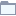# Decision Tree

This category contains 12 nodes.

##### Decision Tree Learner

Decision tree induction performed in memory.

##### Decision Tree LearnerDeprecated

Decision tree induction performed in memory.

##### Decision Tree Predictor

Uses an existing decision tree to compute class labels for input vectors.

##### Decision Tree PredictorDeprecated

Uses an existing decision tree to compute class labels for input vectors.

##### Decision Tree To Image

Renders a decision tree view on an image (PNG).

##### Decision Tree to Ruleset

Converts a decision tree model to PMML RuleSet model.

##### PMML Simple Regression Tree PredictorStreamable

Applies regression from a regression tree model stored in PMML format.

##### Simple Regression Tree Learner

Learns a single regression tree.

##### Simple Regression Tree LearnerDeprecated

Learns a single regression tree.

##### Simple Regression Tree PredictorStreamable

Applies regression from a regression tree model.